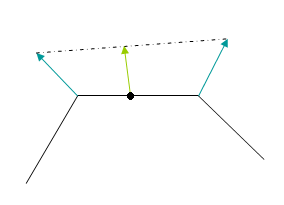Prev: The Normal Matrix Next: Index

When a normal vector arrives at a vertex shader is common to normalize it

`	normal = normalize(gl_NormalMatrix * gl_Normal);`

The multiplication by the gl_NormaMatrix transforms the incoming normal to eye-space. The normalization guarantees a unit length vector as required to compute the cosine with a dot product.

So can we avoid the normalization? We’ll in some cases we can. If the gl_NormaMatrix is orthogonal then we know that the length of the incoming vector is preserved, i.e. the length of normal is equal to the length of gl_Normal. Therefore, if the normals from the OpenGL application are normalized, which is common, we can avoid the normalization in the shader.

In practice this means that if we use gluLookAt to set the camera, and then perform only rotations and translations on our models, we can skip the normalization of the normal vector in the shader. It also means that a directional light will have its direction already normalized.

In the fragment shader we often find ourselves normalizing a vector which was just normalized in the vertex shader. Do we really need to do this? Well, the answer is yes, in most cases we do.

Consider a triangle with three different per vertex normal vectors. The fragment shader receives an interpolated normal, based on the distance from the fragment to the three vertices. The problem is that the interpolated vector, although it has the right direction, it doesn’t have unit length.

The following diagram shows why this is the case. The black lines represent the faces (in 2D), the normals at the vertices are represented in blue. The green vector represents an interpolated normal at the fragment (represented with a dot). All interpolated normals will lie the dotted line. As can be seen in the figure, the green vector is smaller than the blue vectors (which are unit length, at least that was my intention 🙂 ).Note that if the vertex normals were not normalized, not only the length would be different from one, but also the direction would be wrong in the general case. Hence, even if a vector isn’t used on a vertex shader, if we need to have it normalized in the fragment shader, we must also normalize it on the vertex shader.

There is however a case when normalization can be skipped in the fragment shader, as long as the vectors per vertex are normalized. This is when the vectors per vertex all share the same direction, i.e. they are equal. The interpolation of such vectors would yield exactly the same vertex as the per vertex vectors, hence normalized (we assumed that the vertex vectors were normalized).

A simple example is when one considers a directional light. The direction is constant for all fragments, so if the direction is previously normalized, we can skip the normalization step in the fragment shader.

 Prev: The Normal Matrix Next: Index

This site uses Akismet to reduce spam. Learn how your comment data is processed.